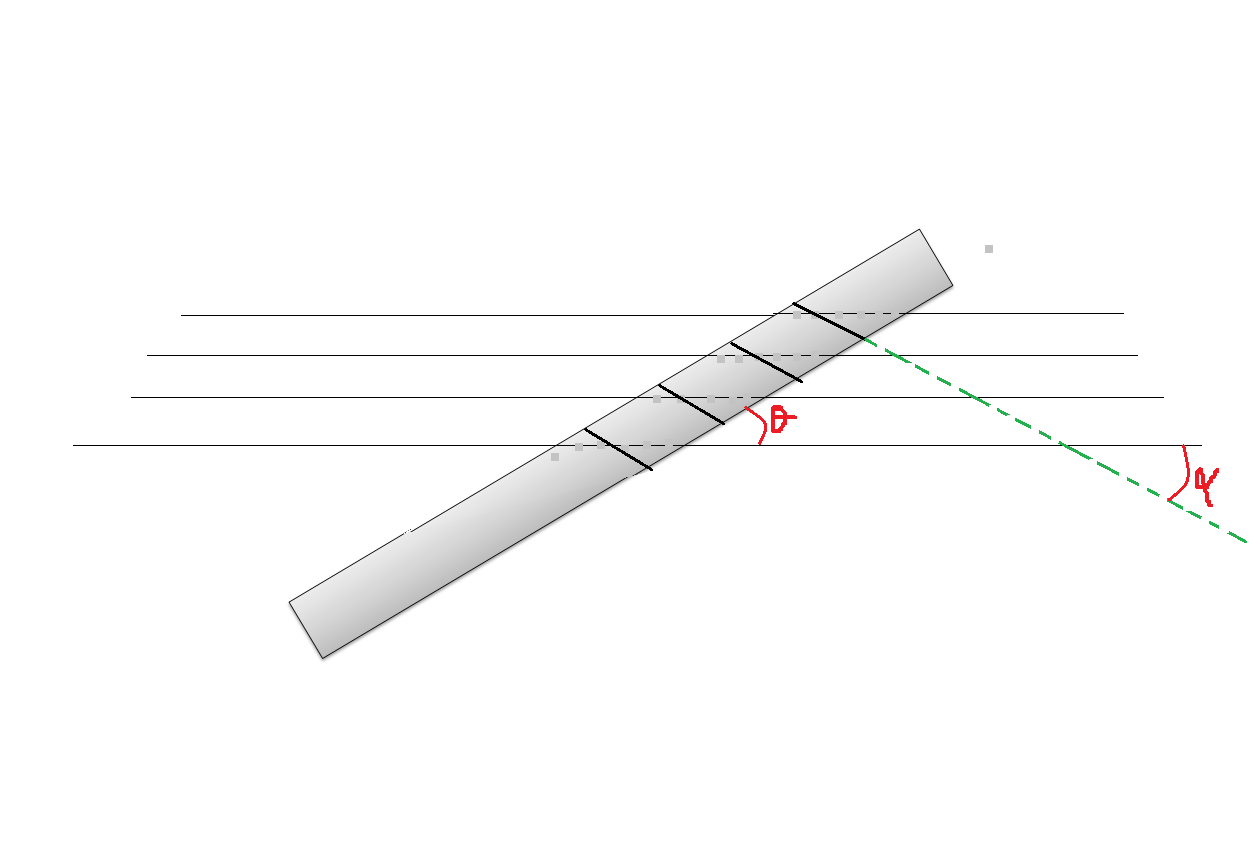# We often see it like thisWe do an experiment with various transparent cylindrical rods made of different refractive indices and place it on ruled white paper of a usual exercise book. If the rod is placed with its axis at some angle $\theta=\frac{\pi}{4}$ the lines appear broken and tilted at some angle $\alpha$ The minimum refractive index of the glass that shows this phenomenon at this angle $\theta=\frac{\pi}{4}$ is $\mu_{o}$ and gives an deviation $\alpha=\alpha_{1}$ find $\mu_{o}\alpha_{1}$ where $\alpha_{1}$is in radian

×